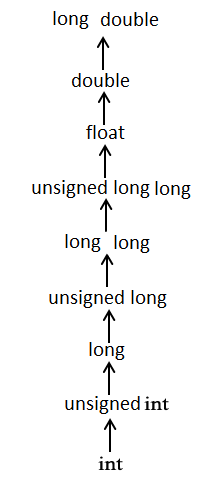# Education is not limited to just classrooms. It can be gained anytime, anywhere... - Ravi Ranjan (M.Tech-NIT)

## Objective-C Type Casting

Type casting is a way to convert a variable from one data type to another data type. For example, if you want to store a long value into a simple integer then you can type cast long to int. You can convert values from one type to another explicitly using the cast operator as follows:

(type_name) expression

In Objective-C, we generally use CGFloat for doing floating point operation, which is derived from basic type of float in case of 32-bit and double in case of 64-bit. Consider the following example where the cast operator causes the division of one integer variable by another to be performed as a floating-point operation:

#import <Foundation/Foundation.h>

int main()
{
int sum = 17, count = 5;
CGFloat mean;

mean = (CGFloat) sum / count;
NSLog(@"Value of mean : %f ", mean );

return 0;
}

When the above code is compiled and executed, it produces the following result:

2013-09-11 01:35:40.047 demo Value of mean : 3.400000

It should be noted here that the cast operator has precedence over division, so the value of sum is first converted to type double and finally it gets divided by count yielding a double value.

Type conversions can be implicit which is performed by the compiler automatically or it can be specified explicitly through the use of the cast operator. It is considered good programming practice to use the cast operator whenever type conversions are necessary.

Integer Promotion

Integer promotion is the process by which values of integer type "smaller" than int or unsigned int are converted either to int or unsigned int. Consider an example of adding a character in an int:

#import <Foundation/Foundation.h>

int main()
{
int  i = 17;
char c = 'c'; /* ascii value is 99 */
int sum;

sum = i + c;
NSLog(@"Value of sum : %d ", sum );

return 0;
}

When the above code is compiled and executed, it produces the following result:

2013-09-11 01:38:28.492 demo Value of sum : 116

Here, value of sum is coming as 116 because compiler is doing integer promotion and converting the value of 'c' to ascii before performing actual addition operation.

Usual Arithmetic Conversion

The usual arithmetic conversions are implicitly performed to cast their values in a common type. Compiler first performs integer promotion, if operands still have different types then they are converted to the type that appears highest in the following hierarchy:The usual arithmetic conversions are not performed for the assignment operators, nor for the logical operators && and ||. Let us take following example to understand the concept:

#import <Foundation/Foundation.h>

int main()
{
int  i = 17;
char c = 'c'; /* ascii value is 99 */
CGFloat sum;

sum = i + c;
NSLog(@"Value of sum : %f ", sum );
return 0;
}

When the above code is compiled and executed, it produces the following result: